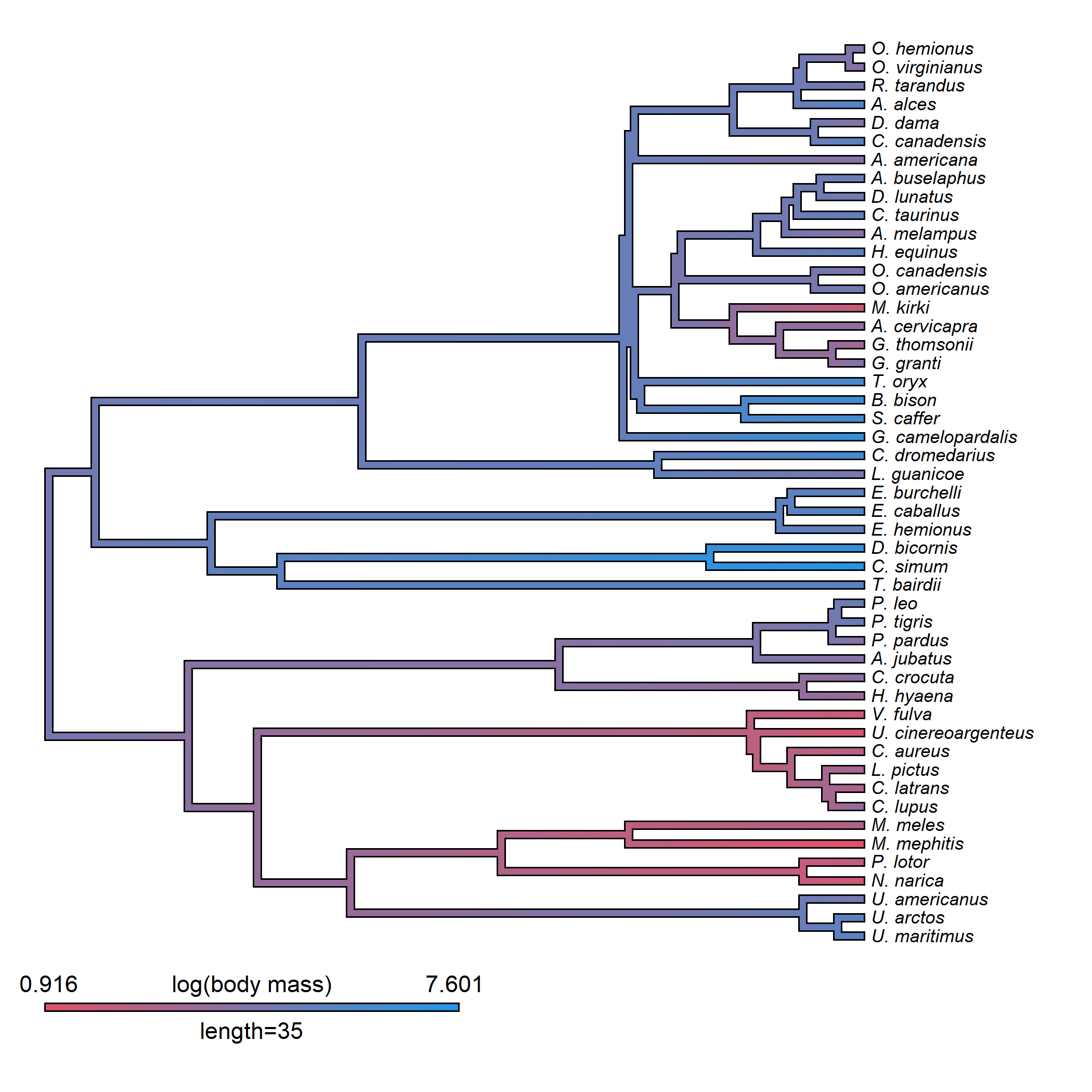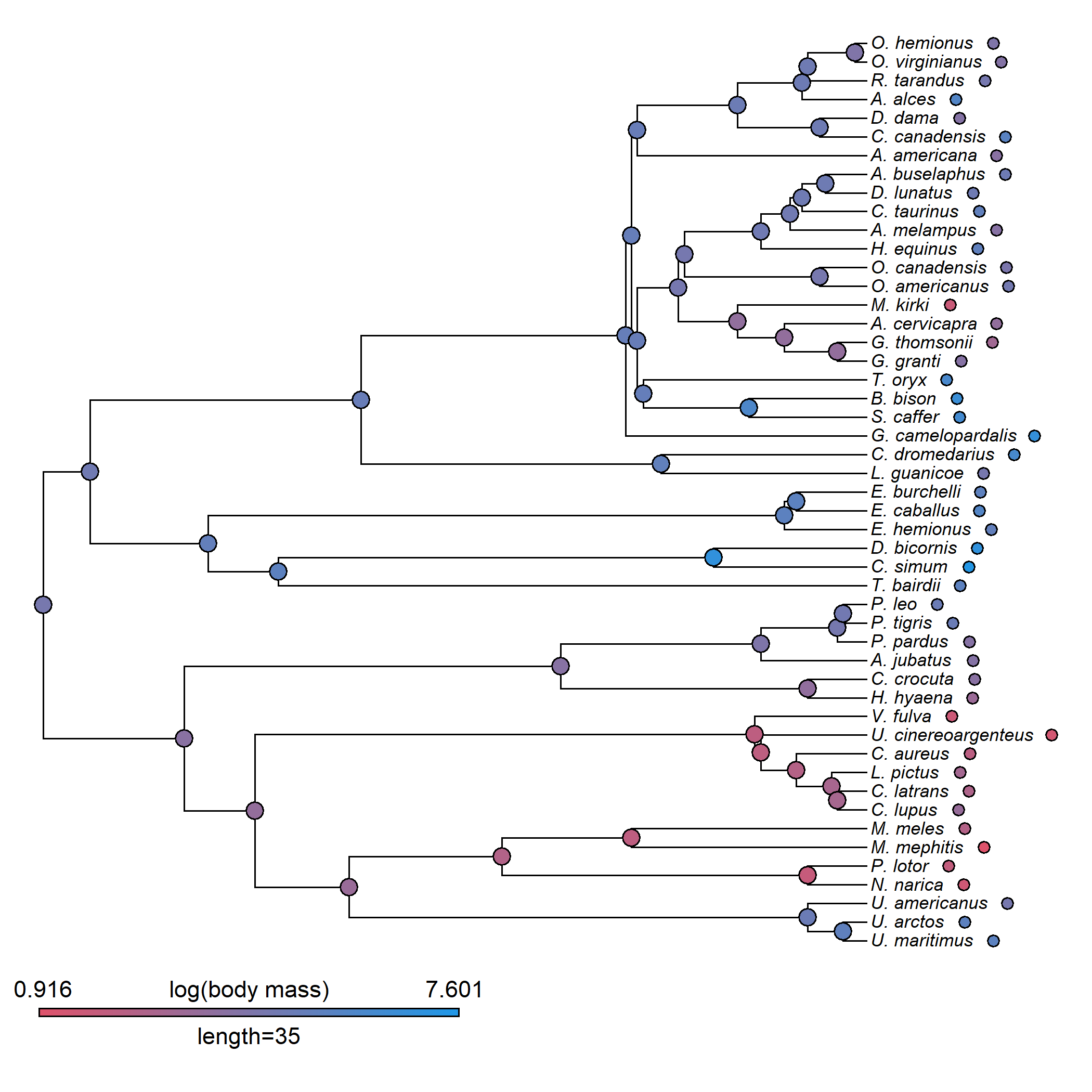## Monday, March 6, 2023

### Mapping the value of a continuous trait at only the nodes of a plotted phylogenetic tree using `contMap`

Inspired by some visualizations during our recent R phylogenetics workshop at UMass-Boston…

… as well as by similar plots in the recent scientific literature…

… I decided to add a simple `nodes_only=TRUE` option to the S3 `plot.contMap` method for the phytools `"contMap"` object class. Updated R code can be seen here.

Here’s how it works.

First, load phytools (updated from GitHub).

``````library(phytools)
packageVersion("phytools")
``````
``````##  '1.5.11'
``````

Now, load a dataset. Here, I’ll use the mammal tree & body mass data from Garland et al. (1992).

``````data(mammal.tree)
data(mammal.data)
lnBodyMass<-setNames(log(mammal.data\$bodyMass),
rownames(mammal.data))
``````

We can next create a `"contMap"` object as follows.

Here is a typical visualization.

``````mammal.contMap<-contMap(mammal.tree,lnBodyMass,plot=FALSE)
mammal.contMap<-setMap(mammal.contMap,palette()[c(2,4)])
plot(mammal.contMap,fsize=c(0.7,0.9),ftype="i",
leg.txt="log(body mass)")
``````Now let’s test the `nodes_only=TRUE` option.

``````plot(mammal.contMap,nodes_only=TRUE,fsize=c(0.7,0.9),
ftype="i",leg.txt="log(body mass)")
``````So far so good.

The implementation works with trees of different types and orientation. Here is a “fan” style tree plot.

``````data(anoletree)
data(anole.data)
svl<-setNames(anole.data\$SVL,rownames(anole.data))
anole.contMap<-contMap(anoletree,svl,plot=FALSE)
anole.contMap<-setMap(anole.contMap,viridisLite::viridis(10))
plot(anole.contMap,nodes_only=TRUE,fsize=c(0.7,0.8),
ftype="i",leg.txt="log(SVL)",type="fan",legend=5,
offset=2)
``````